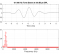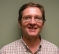## Cross Correlation with Increasing Finite functionsStarted by 6 years ago8 replieslatest reply 6 years ago153 views

I am trying to use cross correlation to find the time delay between two signals. Both signals follow a logarithmic growth pattern, but are sampled for the same amount of time and at the same sample rate. The only difference between the two is: 1) random noise, and 2) the time delay of when the delayed signal starts. When trying traditional cross correlation here it is failing to find the shift and only reports a maximum at zero delay. Please see the attached matlab code for full details

I believe I know why this is, I am just unsure about how to fix it. As the non-delayed signal is slid through all possible delays, the large-valued logarithmic tail goes from being multiplied by another, time-delayed logarithmic function (as is the case when shift = 0) to being multiplied partially by zeros. This ensures that the correlation intensity will not be greater when both signals truly overlap.

Any comments or suggestions would be greatly appreciated

[ - ]So, the two signals (red and blue curves) shown in the figures produced by your script-file will always have the same general shape and offset value (6, in this case)?

Could you use a threshold function to set values above a given value to something larger than 6 prior to computing the correlation?

[ - ]This is an incredibly crafty solution! That actually works, after a fashion. It does not succeed in giving me a peak, but it puts a very clearly differentiable "knee" in the curve at the appropriate position. All I did was threshold above the noise and add a large value. Drowns out the increasing values in the curves themselves and accentuates the rising edge.

Thank you very much!

[ - ]You have to window the 1st function.  In other words, limit the number of samples of your reference waveform.  Then slide that across the waveform you are measuring.  The measured (delayed) waveform has to be long enough that you are not correlating zeros.

[ - ]Thank you so much for taking a look! I tried implementing that, and it seems to work better than before, but there is still not a well defined peak occurring at the position of overlap. Because the functions are both increasing, correlating points beyond the correct alignment continues to yield up larger values. I even tried to zero-pad the template curve. The code below illustrates this:

crossCorrelation.m

correlate.m

Perhaps correlation is not even the correct approach here?

[ - ]Sorry, but cross correlation is the wrong approach here.

When you say "follow a logarithmic growth pattern" I assume that you mean that x(t) = a log(kt), and by "time delay" I assume that you mean y(t) = a log (kt + t0).

Since log(a+b) = log (a(1+b/a)) = log a + log (1+b/a), the difference between your two signals is a log(1 + t0/kt) which approaches log(1) = 0 as t grows.

Y(J)S

[ - ]Yes, I have indeed discovered this. The approach does not work particularly well for my application. Can you recommend an alternative approach?

[ - ]One obvious approach already proposed would be to reduce the noise level by FIR filtering and then finding where a threshold was crossed by each.

A related approach would be to lms fit the two signals to the expected functional form (e.g., a log (t - to)) and compare the arguments of the log.

A slightly different variant would be to build a Wiener filter detector.

Y(J)S

[ - ]Here's a suggestion building on what Y(J)S did:

x(t) = a log( k*t + d_x )
y(t) = a log( k*t + d_y )

Estimate "a".

X(t) = exp( x(t)/a ) ~=~ k*t + d_x
Y(t) = exp( y(t)/a ) ~=~ k*t + d_y

D(t) = X(t) - Y(t) ~=~ d_x - d_y = delay

The best "a" will give the most level D(t) with the best fit to being linear.

Hope it helps,

Ced